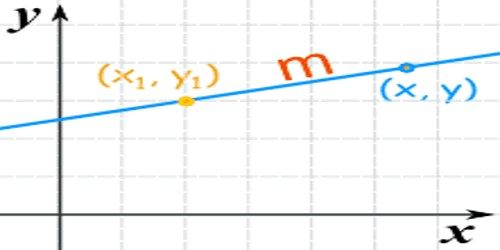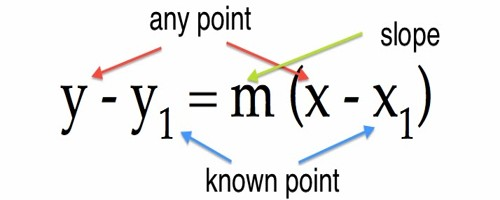Mathematic

# Point-slope Form of a LinePoint-slope Form of a Line

Slope-intercept form is useful when we know the y- intercept of a line. However, we are not always given this information. When we know the slope and one point which is not the y -intercept, we can write the equation in point-slope form.

To find the equation of a straight line passing through a fixed point and having a given slope,

Let AB be the line passing through the point (x1, y1), and let the line be inclined at an angle θ with the positive direction of the x-axis.

Then, tan θ = m = slope.

Let the equation of the line be y = mx + c, ……………. (i)

where m is the slope of the line and c is the y-intercept. As A (x1, y1) is a point on the line AB (x1, y1) satisfy (i).

Therefore, y1 = mx1 + c …………………. (ii)

Subtracting (ii) from (i)

y – y1 = m(x – x1)

The equation of a line passing through(x1, y1) and having the slope m is y – y1 = m(x – x1).

The equation is useful when we know:

• one point on the line
• and the slope of the line, and want to find other points on the line.

For example:

The equation of a line passing through the point (0, 1) and inclined at 30° with the positive direction of the x-axis is y – 1 = tan 30° ∙ (x – 0) or y – 1 = x/√3Remember:

(i) Equation of the y-axis:

The y-axis passes through the origin (0,0) and inclined at 90° with the positive direction of the x-axis.

So, the equation of the y-axis is y – 0 = tan 90° ∙ (x – 0)

⟹ y = ∞ ∙ x

⟹ y/∞ = x

⟹ x = 0

The coordinate of any point on the y-axis is (0, k), where k changes from point to point. Thus, the x-coordinate of any point on the y-axis is 0 and so the equation x = 0 is satisfied by the coordinates of any point on the y-axis. Therefore, the equation of the y-axis is x = 0.

(ii) Equation of a line parallel to the y-axis:

Let AB be a line parallel to the y-axis. Let the line be at a distance a from the y-axis. Then, the slope = tan 90° = ∞ and the line passes through the point (a, 0).

Therefore, the equation of AB is y – 0 = tan 90° ∙ (x – a)

or, y cot 90° = x – a

⟹ y × 0 = x – a

⟹ x – a = 0

⟹ x = a

Information Source: